## Windows command Glossary

Her I am going to report all windows command that I have been used to make easy for me and visitors who would like to return to it at anytime and wherever.

All Windows command will be below I will try to sort it alphabetically:

# D

Listing the files

• dir

Listing all files and directories in the Directory that you are in

• dir /p

Listing all files and sub-directories files

• dir /s

## Dir syntax

Microsoft Windows 2000, XP, Vista, 7, and 8 syntax

Displays a list of files and subdirectories in a directory.

DIR [drive:][path][filename] [/A[[:]attributes]] [/B] [/C] [/D] [/L] [/N] [/O[[:]sortorder]] [/P] [/Q] [/R] [/S] [/T[[:]timefield]] [/W] [/X] [/4]

 [drive:][path][filename] Specifies drive, directory, or files to list. /A Displays files with specified attributes. attributes D- Directories R- Read-only files H- Hidden files A- Files ready for archiving S- System files I- Not content indexed files L- Reparse Points – Prefix meaning not /B Uses bare format (no heading information or summary). /C Display the thousand separator in file sizes. This is the default. Use /-C to disable display of separator. /D Same as wide but files are list sorted by column. /L Uses lowercase. /N New long list format where filenames are on the far right. /O List by files in sorted order. sortorder N By name (alphabetic) S By size (smallest first) E By extension (alphabetic) D By date/time (oldest first) G Group directories first – Prefix to reverse order /P Pauses after each screenful of information. /Q Display the owner of the file. /R Display alternate data streams of the file. /S Displays files in specified directory and all subdirectories. /T Control what time field displayed or used for sorting timefield C Creation A Last Access W Last Written /W Uses wide list format. /X This displays the short names generated for non-8dot3 file names. The format is that of /N with the short name inserted before the long name. If no short name is present, blanks are displayed in its place. /4 Displays four-digit years

Microsoft Windows 95, 98, and ME syntax

Displays a list of files and subdirectories in a directory.

DIR [drive:][path][filename] [/P] [/W] [/A[[:]attributes]] [/O[[:]sortorder]] [/S] [/B] [/L] [/V]

 [drive:][path][filename] Specifies drive, directory, or files to list. (Could be enhanced file specification ormultiple filespecs.) /P Pauses after each screenful of information. /W Uses wide list format. /A attributes: D Directories R Read-only files H Hidden files A Files ready for archiving S System files – Prefix meaning not /O List by files in sorted order, sortorder: N By name (alphabetic) S By size (smallest first) E By extension (alphabetic) D By date and time (earliest first) G Group directories first – Prefix to reverse order A By Last Access Date (earliest first) /S Displays files in specified directory and all subdirectories. /B Uses bare format (no heading information or summary). /L Uses lowercase. /V Verbose mode.

Switches may be preset in the DIRCMD environment variable. Override preset switches by prefixing any switch with – (hyphen)–for example, /-W.

## Dir examples

dir

Lists all files and directories in the current directory. By default the dir command lists the files and directories in alphabetic order.

dir *.exe

The above command lists any file that ends with the .exe file extension. See the wildcarddefinition for further wildcard examples.

dir *.txt *.doc

The above is using multiple filespecs to list any files ending with .txt and .doc in one command.

List only the directories in the current directory. If you need to move into one of the directories listed use the cd command.

dir /s

Lists the files in the directory that you are in and all sub directories after that directory, if you are at root “C:\>” and type this command this will list to you every file and directory on the C: drive of the computer.

dir /p

If the directory has lots of files and you cannot read all the files as they scroll by, you can use this command and it displays all files one page at a time.

dir /w

If you don’t need file information you can use this command to list only the files and directories going horizontally, taking as little as space needed.

dir /s /w /p

This would list all the files and directories in the current directory and the sub directories after that, in wide format and one page at a time.

dir /on

List the files in alphabetical order by the names of the files.

dir /o-n

List the files in reverse alphabetical order by the names of the files.

dir \ /s |find “i” |more

A nice command to list all directories on the hard drive, one screen page at a time, and see the number of files in each directory and the amount of space each occupies.

dir > myfile.txt

Takes the output of dir and re-routes it to the file myfile.txt instead of outputting it to the screen.

# N

Used for:

• Finding computer name using IP

# Z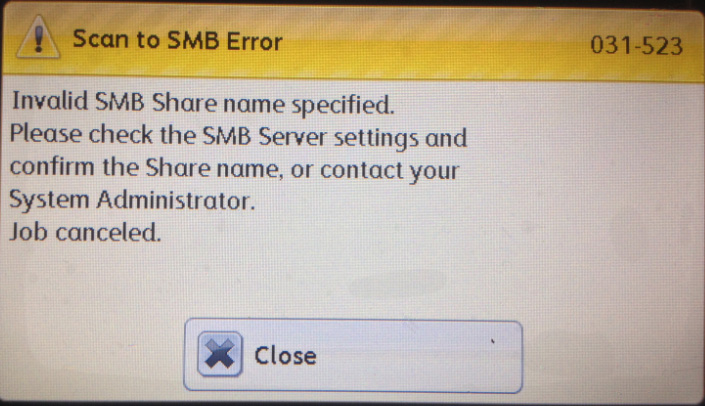## scan to smb error 031-523

source

I was trying to configure network scanning on a brand new Xerox WorkCenter 6605 network printer. I managed to specify the SMB details via printer’s web interface but despite hours of playing with different configuration settings I was unable to get the network scan working.

Here is the Error

Error No. 031-523
Scan To SMB Error – Invalid SMB Share Name Specified.

I spent about 3 hours in trying with different values and combination for “Login Name (if required by host):“, “Name of Shared Directory:” and “Subdirectory Path (optional):” but none of these combinations worked.

I contacted Xerox support which was picked up by a girl in Philippines. While still on phone with her and trying to play around with the values I managed to get it working with the following settings:

This setting will give you a path which you can enter in Windows Explorer and able to see the folder.
In my case if I copy \\192.168.254.10\Users Share\Scanned Documents and pasted in windows explorer I’m able to see the folder’s content.

So the trick here is to first identify your host by IP address, then find a top level folder and finally a sub folder (optional).

Avoid entering any forward or backward slashes in any of these two folder paths.

The biggest pain was figuring out the login name. After some search I found confusing answers. In one Xerox forum posts it was mentioned to specify DOMAIN/username and on a Microsoft support page they mentioned DOMAIN\username – pay attention to the backward and forward slashes. For me it’s just theusername worked.

Once I figured that out the next problem was finding the path for folders. I was making mistakes in adding slashes before the folder names which is not required.

And as you might have already guessed it is a Windows Network (Windows 2003 to be precise).

Here is the visuals of the shared scanned folder and how to map it to the Xerox WorkCenter 6605 SMB scan settings.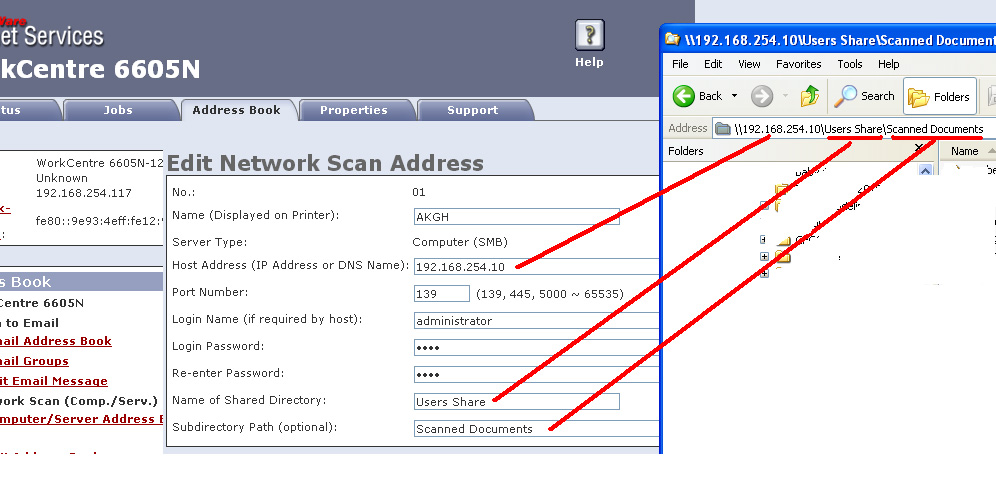Xerox WorkCenter 6605, Scan To SMB Error, Invalid SMB Share Name Specified,031-523,Xerox WorkCenter 6605 SMB scan settings vs Network path## Show or Hide HDD icon on desktop of mac

Show or Hide HDD icon on desktop of mac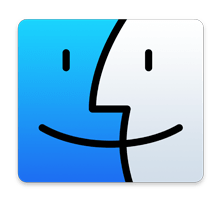You can easily hide or show the “Macintosh HD” main hard drive from the desktop of OS X, along with any other internal volumes and removable drives by adjusting some Finder options.

If you like to keep your disk drives available on the desktop for easy access like this, here’s what you can do to be sure they’re always visible on the Mac desktop:

### How to Show (or Hide) Hard Drives, Disks, and Volumes on the Mac OS X Desktop

This feature is available in all versions of OS X:

1. Go to the desktop of the Mac if you haven’t yet
2. Launch the Finder Preferences from the “Finder” menu, or hit Command+,
3. Under the ‘General’ tab, check or uncheck items you either want shown or hidden, respectively
4. Close Finder preferences

The settings look like the following: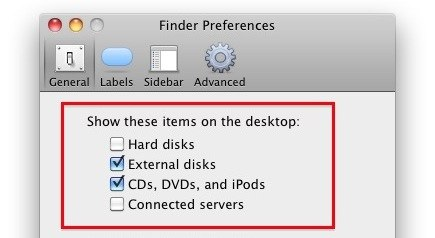These changes take effect immediately. Through these preferences you can adjust the visibility of Macintosh HD and other internal hard disks, external drives, CD’s, DVD’s, iPods, and even connected servers.

Macintosh HD is categorized under ‘Hard disks’ so if you leave it checked it will remain visible.

Each drive will have a unique icon visible on the desktop.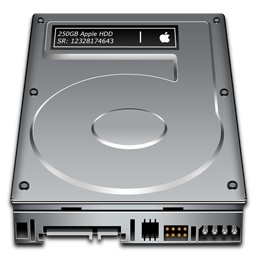If you’re a minimalist or you just don’t like the desktop icons and the clutter they can cause, you can always hide all desktop icons in Mac OS X with a simple Terminal command as well.

## Number Converting

Number Converting

Source: http://www.robotroom.com/

# Number Systems

There are infinite ways to represent a number. The four commonly associated with modern computers and digital electronics are: decimal, binary, octal, and hexadecimal.

Decimal (base 10) is the way most human beings represent numbers. Decimal is sometimes abbreviated as dec.

Decimal counting goes:
0, 1, 2, 3, 4, 5, 6, 7, 8, 9, 10, 11, 12, 13, 14, 15, 16, 17, 18, and so on.

Binary (base 2) is the natural way most digital circuits represent and manipulate numbers. (Common misspellings are “bianary”, “bienary”, or “binery”.) Binary numbers are sometimes represented by preceding the value with ‘0b’, as in 0b1011. Binary is sometimes abbreviated as bin.

Binary counting goes:
0, 1, 10, 11, 100, 101, 110, 111, 1000, 1001, 1010, 1011, 1100, 1101, 1110, 1111, 10000, 10001, and so on.

Octal (base 8) was previously a popular choice for representing digital circuit numbers in a form that is more compact than binary. Octal is sometimes abbreviated as oct.

Octal counting goes:
0, 1, 2, 3, 4, 5, 6, 7, 10, 11, 12, 13, 14, 15, 16, 17, 20, 21, and so on.

Hexadecimal (base 16) is currently the most popular choice for representing digital circuit numbers in a form that is more compact than binary. (Common misspellings are “hexdecimal”, “hexidecimal”, “hexedecimal”, or “hexodecimal”.) Hexadecimal numbers are sometimes represented by preceding the value with ‘0x’, as in 0x1B84. Hexadecimal is sometimes abbreviated as hex.

0, 1, 2, 3, 4, 5, 6, 7, 8, 9, A, B, C, D, E, F, 10, 11, and so on.

All four number systems are equally capable of representing any number. Furthermore, a number can be perfectly converted between the various number systems without any loss of numeric value.

At first blush, it seems like using any number system other than human-centric decimal is complicated and unnecessary. However, since the job of electrical and software engineers is to work with digital circuits, engineers require number systems that can best transfer information between the human world and the digital circuit world.

It turns out that the way in which a number is represented can make it easier for the engineer to perceive the meaning of the number as it applies to a digital circuit. In other words, the appropriate number system can actually make things less complicated.

## Fundamental Information Element of Digital Circuits

Almost all modern digital circuits are based on two-state switches. The switches are either on or off. It doesn’t matter if the switches are actually physical switches, vacuum tubes, relays, or transistors. And, it doesn’t matter if the ‘on’ state is represented by 1.8 V on a cutting-edge CPU core, -12 V on a RS-232 interface chip, or 5 V on a classic TTL logic chip.

Because the fundamental information element of digital circuits has two states, it is most naturally represented by a number system where each individual digit has two states: binary.

For example, switches that are ‘on’ are represented by ‘1’ and switches that are ‘off’ are represented by ‘0’. It is easy to instantly comprehend the values of 8 switches represented in binary as 10001101. It is also easy to build a circuit to display each switch state in binary, by having an LED (lit or unlit) for each binary digit.

## Making Values More Compact

“Binary digit” is a little unwieldy to say over and over, so the term was contracted to “bit”. Not only is the term “binary digit” a little unwieldy, but so is the length of a binary number, since each digit can only represent one switch. As digital circuits grew more complex, a more compact form of representing circuit information became necessary.

An octal number (base 8) can be up to 1/3 the length of a binary number (base 2). 8 is a whole power of 2 (23=8). That means three binary digits convert neatly into one octal digit.

A hexadecimal number (base 16) can be up to 1/4 the length of a binary number. 16 is a whole power of 2 (24=16). That means four binary digits convert neatly into one hexadecimal digit.

Unfortunately, decimal (base 10) is not a whole power of 2. So, it is not possible to simply chunk groups of binary digits to convert the raw state of a digital circuit into the human-centric format.

Let’s see how to convert a number from binary.

[incor name=”2. Binary Number Conversion”]

# 2. Binary Number Conversion

## Binary to Octal

An easy way to convert from binary to octal is to group binary digits into sets of three, starting with the least significant (rightmost) digits.

 Binary: 11100101 = 11 100 101 011 100 101 Pad the most significant digits with zeros if necessary to complete a group of three.

Then, look up each group in a table:

 Binary: 000 001 010 011 100 101 110 111 Octal: 0 1 2 3 4 5 6 7

 Binary = 011 100 101 Octal  = 3 4 5 = 345 oct

An equally easy way to convert from binary to hexadecimal is to group binary digits into sets of four, starting with the least significant (rightmost) digits.

Binary: 11100101 = 1110 0101

Then, look up each group in a table:

 Binary: 0000 0001 0010 0011 0100 0101 0110 0111 Hexadecimal: 0 1 2 3 4 5 6 7
 Binary: 1000 1001 1010 1011 1100 1101 1110 1111 Hexadecimal: 8 9 A B C D E F

 Binary = 1110 0101 Hexadecimal = E 5 = E5 hex

## Binary to Decimal

They say there are only 10 people in this world: those that understand binary and those that don’t. Ha ha.

If you don’t get that joke, you’ll need a method to convert from binary to decimal. One method involves addition and multiplication.

1. Start the decimal result at 0.
2. Remove the most significant binary digit (leftmost) and add it to the result.
3. If all binary digits have been removed, you’re done. Stop.
4. Otherwise, multiply the result by 2.
5. Go to step 2.

Here is an example of converting 11100000000 binary to decimal:

 Binary Digits Operation Decimal Result Operation Decimal Result 11100000000 +1 1 × 2 2 1100000000 +1 3 × 2 6 100000000 +1 7 × 2 14 00000000 +0 14 × 2 28 0000000 +0 28 × 2 56 000000 +0 56 × 2 112 00000 +0 112 × 2 224 0000 +0 224 × 2 448 000 +0 448 × 2 896 00 +0 896 × 2 1792 0 +0 1792 done.

Let’s try converting from decimal

# 3. Decimal Number Conversion

A repeated division and remainder algorithm can convert decimal to binary, octal, or hexadecimal.

1. Divide the decimal number by the desired target radix (2, 8, or 16).
2. Append the remainder as the next most significant digit.
3. Repeat until the decimal number has reached zero.

## Decimal to Binary

Here is an example of using repeated division to convert 1792 decimal to binary:

 Decimal Number Operation Quotient Remainder Binary Result 1792 ÷ 2 = 896 0 0 896 ÷ 2 = 448 0 00 448 ÷ 2 = 224 0 000 224 ÷ 2 = 112 0 0000 112 ÷ 2 = 56 0 00000 56 ÷ 2 = 28 0 000000 28 ÷ 2 = 14 0 0000000 14 ÷ 2 = 7 0 00000000 7 ÷ 2 = 3 1 100000000 3 ÷ 2 = 1 1 1100000000 1 ÷ 2 = 0 1 11100000000 0 done.

### Decimal to Octal

Here is an example of using repeated division to convert 1792 decimal to octal:

 Decimal Number Operation Quotient Remainder Octal Result 1792 ÷ 8 = 224 0 0 224 ÷ 8 = 28 0 00 28 ÷ 8 = 3 4 400 3 ÷ 8 = 0 3 3400 0 done.

Here is an example of using repeated division to convert 1792 decimal to hexadecimal:

 Decimal Number Operation Quotient Remainder Hexadecimal Result 1792 ÷ 16 = 112 0 0 112 ÷ 16 = 7 0 00 7 ÷ 16 = 0 7 700 0 done.

The only addition to the algorithm when converting from decimal to hexadecimal is that a table must be used to obtain the hexadecimal digit if the remainder is greater than decimal 9.

 Decimal: 0 1 2 3 4 5 6 7 Hexadecimal: 0 1 2 3 4 5 6 7
 Decimal: 8 9 10 11 12 13 14 15 Hexadecimal: 8 9 A B C D E F

The addition of letters can make for funny hexadecimal values. For example, 48879 decimal converted to hex is:

 Decimal Number Operation Quotient Remainder Hexadecimal Result 48879 ÷ 16 = 3054 15 F 3054 ÷ 16 = 190 14 EF 190 ÷ 16 = 11 14 EEF 11 ÷ 16 = 0 11 BEEF 0 done.

Now on to octal conversions…

# 4. Octal Number Conversion

## Octal to Binary

Converting from octal to binary is as easy as converting from binary to octal. Simply look up each octal digit to obtain the equivalent group of three binary digits.

 Octal: 0 1 2 3 4 5 6 7 Binary: 000 001 010 011 100 101 110 111

 Octal  = 3 4 5 Binary = 011 100 101 = 011100101 binary

When converting from octal to hexadecimal, it is often easier to first convert the octal number into binary and then from binary into hexadecimal. For example, to convert 345 octal into hex:

(from the previous example)

 Octal  = 3 4 5 Binary = 011 100 101 = 011100101 binary

Drop any leading zeros or pad with leading zeros to get groups of four binary digits (bits):
Binary 011100101 = 1110 0101

Then, look up the groups in a table to convert to hexadecimal digits.

 Binary: 0000 0001 0010 0011 0100 0101 0110 0111 Hexadecimal: 0 1 2 3 4 5 6 7
 Binary: 1000 1001 1010 1011 1100 1101 1110 1111 Hexadecimal: 8 9 A B C D E F

 Binary = 1110 0101 Hexadecimal = E 5 = E5 hex

Therefore, through a two-step conversion process, octal 345 equals binary 011100101 equals hexadecimal E5.

## Octal to Decimal

Converting octal to decimal can be done with repeated division.

1. Start the decimal result at 0.
2. Remove the most significant octal digit (leftmost) and add it to the result.
3. If all octal digits have been removed, you’re done. Stop.
4. Otherwise, multiply the result by 8.
5. Go to step 2.
 Octal Digits Operation Decimal Result Operation Decimal Result 345 +3 3 × 8 24 45 +4 28 × 8 224 5 +5 229 done.

The conversion can also be performed in the conventional mathematical way, by showing each digit place as an increasing power of 8.

345 octal = (3 * 82) + (4 * 81) + (5 * 80) = (3 * 64) + (4 * 8) + (5 * 1) = 229 decimal

Converting from hexadecimal to binary is as easy as converting from binary to hexadecimal. Simply look up each hexadecimal digit to obtain the equivalent group of four binary digits.

 Hexadecimal: 0 1 2 3 4 5 6 7 Binary: 0000 0001 0010 0011 0100 0101 0110 0111
 Hexadecimal: 8 9 A B C D E F Binary: 1000 1001 1010 1011 1100 1101 1110 1111

 Hexadecimal = A 2 D E Binary = 1010 0010 1101 1110 = 1010001011011110 binary

When converting from hexadecimal to octal, it is often easier to first convert the hexadecimal number into binary and then from binary into octal. For example, to convert A2DE hex into octal:

(from the previous example)

 Hexadecimal = A 2 D E Binary = 1010 0010 1101 1110 = 1010001011011110 binary

Binary: 1010001011011110 = 001 010 001 011 011 110

Then, look up each group in a table:

 Binary: 000 001 010 011 100 101 110 111 Octal: 0 1 2 3 4 5 6 7

 Binary = 001 010 001 011 011 110 Octal = 1 2 1 3 3 6 = 121336 octal

Therefore, through a two-step conversion process, hexadecimal A2DE equals binary 1010001011011110 equals octal 121336.

Converting hexadecimal to decimal can be performed in the conventional mathematical way, by showing each digit place as an increasing power of 16. Of course, hexadecimal letter values need to be converted to decimal values before performing the math.

 Hexadecimal: 0 1 2 3 4 5 6 7 Decimal: 0 1 2 3 4 5 6 7 Hexadecimal: 8 9 A B C D E F Decimal: 8 9 10 11 12 13 14 15

= ((A) * 163) + (2 * 162) + ((D) * 161) + ((E) * 160)
= (10 * 163) + (2 * 162) + (13 * 161) + (14 * 160)
= (10 * 4096) + (2 * 256) + (13 * 16) + (14 * 1)
= 40960 + 512 + 208 + 14
= 41694 decimal

Finally, why you might choose one number system over another…

# 6. Selecting a Numbering System

The appropriate numbering system to represent a value depends on the application. There are advantages and disadvantages to each numbering system.

When interfacing with non-engineers, decimal remains the numbering system of choice. For example, I’d have heart attack if the IRS told me that I owed them 1000000 dollars, when they actually meant to say 64 decimal.

Modern computers have lots of memory and disk space for programs. However, many low-end microcontrollers can be quite tight on space. Outputting a decimal value to a serial port or display requires the number be divided by 10 to break off each digit. Depending on the capabilities of the microcontroller, the divide-by-ten routine alone can take up more room than an entire hexadecimal output routine!

When diagnosing an output pin logic problem, it isn’t immediately helpful to see that the microcontroller’s port B is set to 180 decimal. I’d prefer to see the pins as 10110100 binary. The same thing is true when looking at bit flags in memory. A disadvantage of binary is that it gets lengthy fairly quickly.

When debugging dumps of memory, disks, or data streams on modern microcontroller and computers, hexadecimal provides a compact format that is easy to break into bytes and convert into bits. In my software career, I routine examine lengthy log files detailing all the bytes sent over the air on the wireless networks.

Octal has fallen out of usage over the last thirty years due to microprocessors and microcontrollers processing data in bit chunks that are not evenly divisible by 3 (4-bit, 8-bit, 16-bit, 32-bit, 64-bit) . Octal does have the advantage of requiring only number symbols (0-8), as opposed to hexadecimal (0-9, A-F). So, octal still finds some limited usage on numeric-only displays.

To summarize, I switch between using binary, decimal, and hexadecimal on a regular basis, depending which format most clearly expresses the data that the number represents.

# CCNP ROUTE Practice Exam:

Multiarea OSPF 4

Chris Bryant, “The Computer Certification Bulldog”

Here’s the 4th practice exam in this series. Dig in!

Question 1:

You’re configuring an OSPF ASBR, and have determined that all the routes injected into OSPF via redistribution can be summarized with the address 23.0.0.0 /12.

What is the full OSPF command to advertise this summary into the OSPF domain in place of the specific routes?

Question 2:

You’re performing two-way route redistribution between an EIGRP AS and an OSPF domain. On the OSPF routers, what is the default routing table code for the routes learned from EIGRP?

Extra credit: What code will appear on the EIGRP routers next to any routes that are injected into the EIGRP AS from OSPF?

Consider the following OSPF configuration and answer the following questions.

R4(config)#router ospf 1

R4(config-router)#network 172.12.0.0 0.0.0.255 area 0

R4(config-router)#network 1.1.1.1 0.0.0.0 area 1

R4(config-router)#router-id 20.1.1.1

< router reloaded at this point >

R4(config)#interface loopback0

Question 3:

Is that a legal configuration? If not, why not?

Question 4:

What is the OSPF process number?

Question 5:

What is the RID of this particular router?

Question 6:

Is this router an ABR, ASBR, both, or neither?

Explain why this router is or isn’t an ABR.

Explain why this router is or isn’t an ASBR.

This ends the questions about that router configuration.

Question 7:

An OSPF total stub router can contain what route types?

A. Default route only

B. E2 routes and a default route

C. E1 routes and a default route

D. Intra-area routes and a default route

E. Inter-area routes and a default route

1. The full command is:

2. These routes will appear as OSPF External Type 2 routes and will have a router table code of “O E2”.

The routes that are redistributed from OSPF into EIGRP will appear in the EIGRP routing tables with a code of “D EX”.

3. Yep, it’s legal.

4. The OSPF process number is 1, as indicated by the router ospf 1 command.

5. The OSPF RID was hardcoded as 20.1.1.1, and it took effect after the router reload I mentioned.

6. This router is an ABR since it borders Area 1 and Area 0.

There is no route redistribution shown on this router, so it’s not an ASBR.

7. “D”. The routing table of a total stub router can contain intra-area routes and a default inter-area route using the ABR as the next hop.

Thanks for taking this CCNP practice exam – here are the other OSPF multiarea exams!